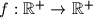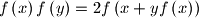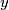### IMO Shortlist 2005 problem A2

Kvaliteta:
Avg: 3.0
Težina:
Avg: 6.0
We denote by$\mathbb{R}^+$ the set of all positive real numbers.

Find all functions$f: \mathbb R^ + \rightarrow\mathbb R^ +$ which have the property:for all positive real numbers$x$ and$y$.
Source: Međunarodna matematička olimpijada, shortlist 2005